Next: Isospin structure of the Up: Theory Previous: Isospin projection formalism

## Inverse of the overlap matrix

A prerequisite for the calculation of the integrals in Eqs. (2), (10), and (13) is the isospin rotation of the Slater determinant. To this end, one has to perform an independent rotation of s.p. neutron,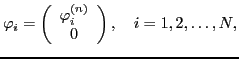(19)

and proton,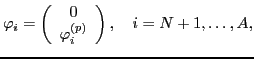(20)

HF wave functions. The use of the two-dimensional (spinor) representation of isospin constitutes a natural formalism for the isospin projection technique. In this representation, the upper (neutron,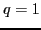) and lower (proton,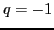) components of the isospin-rotated states are given by: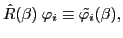(21)

where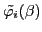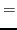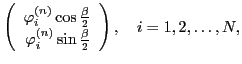(22)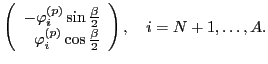(23)

To calculate the kernel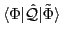of an arbitrary operator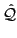between two non-orthogonal Slater determinants, we apply the generalized Wick's theorem, see, e.g., Ref. . In particular, the norm kernel can be written in a compact form: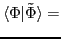Det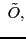(24)

where the overlap matrix is: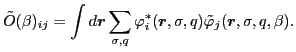(25)

To calculate kernels appearing in the projection formalism, one needs to invert the overlap matrix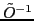. This can cause serious problems due to the presence of singularities [22,40]. The regularization of kernel singularities is a difficult problem . Thus far, a regularization scheme has been worked out only for a very specific class of functionals (or effective density-dependent interactions) solely involving integer powers of local densities . Unfortunately, almost all commonly used Skyrme and Gogny parameterizations, except for SIII , involve fractional powers of the density. The appearance of singularities prevents us from using the local Slater approximation for the Coulomb exchange. In the present work we treat it exactly using the method of the Gaussian decomposition of the Coulomb interaction, as described in Refs. [42,43].

Compared to the particle number or the angular momentum projection schemes, isospin projection is a relatively simple procedure. In particular, the dependence of the inverse matrix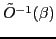on the isorotation angle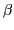can be determined analytically and this enables us to demonstrate that the isospin projection is free from kernel singularities. To this end, we write the overlap matrix (25) in the form: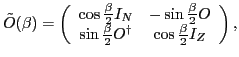(26)

where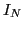(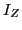) stands for the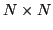(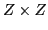) unit matrix, while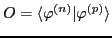is the rectangular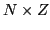overlap matrix of the neutron and proton s.p. wave functions. Using the singular value decomposition (SVD) technique, the matrix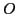can be written as: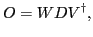(27)

where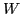is anrectangular matrix having orthogonal columns (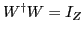),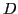is a diagonalreal and non-negative matrix (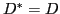), and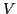is aquadratic unitary matrix (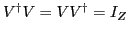). The SVD decomposition (27) further implies that:(28a)

where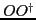and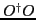are matrices of dimensionand, respectively.

The SVD decomposition allows us to analytically diagonalize the overlap matrix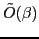. Without loss of generality we assume that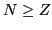. Hence, the product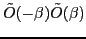can be written as: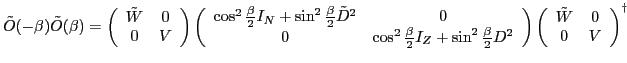(29)

where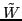of dimensionis a unitary matrix composed of columns: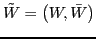where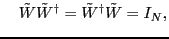(30)

and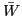is the unitary complement.

The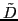matrix, on the other hand, is thematrixcompleted to the dimensionby zeros: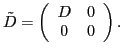(31)

Since the first and third matrices on the right hand side of Eq. (29) are unitary and the second matrix is diagonal, the inverse of the overlap matrix reads: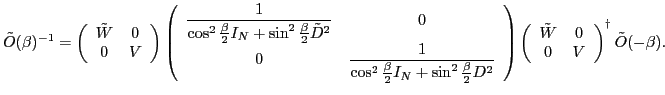(32)

This expression shows explicitly that the inverse of the overlap matrix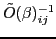can be singular only if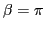. Indeed, in the case of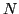=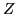, the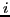th denominator becomes zero,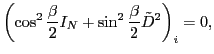(33)

only whenand the corresponding singular value vanishes: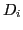=0. In the case of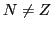, the only difference is that the matrixcontains zeros by construction, see Eq. (31). Fortunately, for the isospin projection, the singularity atis compensated by the Jacobian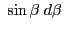.Next: Isospin structure of the Up: Theory Previous: Isospin projection formalism
Jacek Dobaczewski 2010-01-30